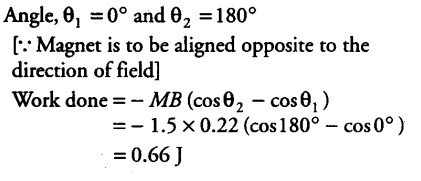# What is the torque on the magnet in cases (a) and (b)?

A bar magnet of magnetic moment 1.5 J/T lies aligned with the direction of a uniform magnetic field of 0.22 T.
(i)What is the amount of work required by an external torque to turn the magnet, so as to align its magnetic moment (a) normal to the field direction, (b) opposite to the field direction?
(ii) What is the torque on the magnet in cases (a) and (b)?

Given, magnetic moment of magnet, M = 1.5 J/T Uniform magnetic field, B = 0.22 T
(i) a) Angle, { θ }_{ 1 } = 0° [ The magnet lies aligned in the direction of field]
and {θ }_{ 2 } = 90° [ The magnet is to be aligned normal to the field]
Work done in rotating the magnet from angle\${ θ }{ 1 } to {θ }{ 2 }\$

W = -MB ( cos\${ θ }{ 1 } - cos {θ }{ 2 }\$ )
= -1.5 x 0.22 ( cos 90° - cos 0° )
=0.33 J
(b)(ii) Using the formula of torque,
τ = MB sinθ
(a) When magnetic moment is normal to the field,
θ= 90°
τ = 1.5 x 0.22 sin 90° = 0.33 N-m
(b) When magnetic moment opposite to the field,
θ= 180°
τ = 1.5 x 0.22 sin 180° = 0.